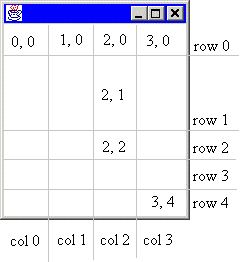TableLayout - An Alternative to GridBagLayout

A Simple and Powerful Layout Manager
by Daniel Barbalace

The TableLayout.jar contains the source code and class files for both TableLayout and all of the examples in this article.Introduction to TableLayout

What is TableLayout?

TableLayout is a layout manager that partitions a container into a set of rows and columns. The intersection of a row and a column is called a cell, like in a spreadsheet. Components added to the container are placed in cells. As the container is resized, the cells are also resized. Consequentially, the components inside those cells are resized.

To illustrate, consider a simple frame divided into four columns and five rows as show in the following illustration.Notice that not all of the rows have the same height. Every row can be assigned a different height. Similarly, every column can be assigned a different width. As the container is resized, every row and column is also resized according to its specification.

 Side Note Anything said about a row also applies to a column and vice versa. For example, whatever you can say about a row's height, you can also say about a column's width. TableLayout is symmetrical in the x and y dimensions. So for brevity, this document will discuss such symmetical issues in the form of columns and horizontal values.

The rows and columns are marked in the illustration. Notice that the first column is column 0. Also notice that the cells are labeled as (column, row). This is anologous to (x, y) coordinates. The upper-leftmost cell is (0, 0) and the bottom-rightmost cell is (3, 4). The gray lines dividing the rows and columns are imaginary. You do not see them in the container.

Creating a TableLayout

The illustration shows the division of the container at a one possible size. What happens when the container is resized? That depends on how the rows and columns are specified. It might be desirable for each column to be given an equal width, or you may prefer the first three columns to be fixed and the fourth column to be given all remaining space.

There a five ways to specify the width of a column. A column can be be given an absolute width in pixels, a percentage of available space, an equal portion of all remaining space, a width determined by the preferred size of the components occupying that column, or a width determined by the minimum size of the components occupying that column.

For the above illustration, let's assume that we want all columns to have an equal width, row 1 to be resized with the container, and all other rows to have a fix size. The following code will create the frame.

 public static void createFrame () { Frame frame = new Frame(); frame.setBounds (100, 100, 300, 300); frame.show(); double size[][] = {{0.25, 0.25, 0.25, 0.25}, {50, TableLayout.FILL, 40, 40, 40}}; frame.setLayout (new TableLayout(size)); }

Notice that creating a TableLayout involves two steps. First, a two-dimensional array of doubles is created to specify the column widths and row heights. Second, the TableLayout itself is created using this array. The length of size is 2. size contains the widths of each column, and size contains the height of each row.

The column widths are specified as 25% or 0.25. All real numbers in the range [0.0..1.0) represents percentages. Notice that the upper end of this interval is open. The value 1.0 does not mean 100%. It means 1 pixel. However, there is a way to give a column 100% of the scalable space. This is discussed below. The value 0.0 can be interpreted as either 0% or 0 pixels.

The first row, row 0, is allocated exactly 50 pixels. All non-negative integers refer to absolute pixel sizes. Rows 2 to 4 are allocated 40 pixels. Row 1, specified with the constant FILL is allocated 100% of the scalable space. The term FILL means to fill this row with the remaining space.

NextJava SDKs and ToolsJava Resources